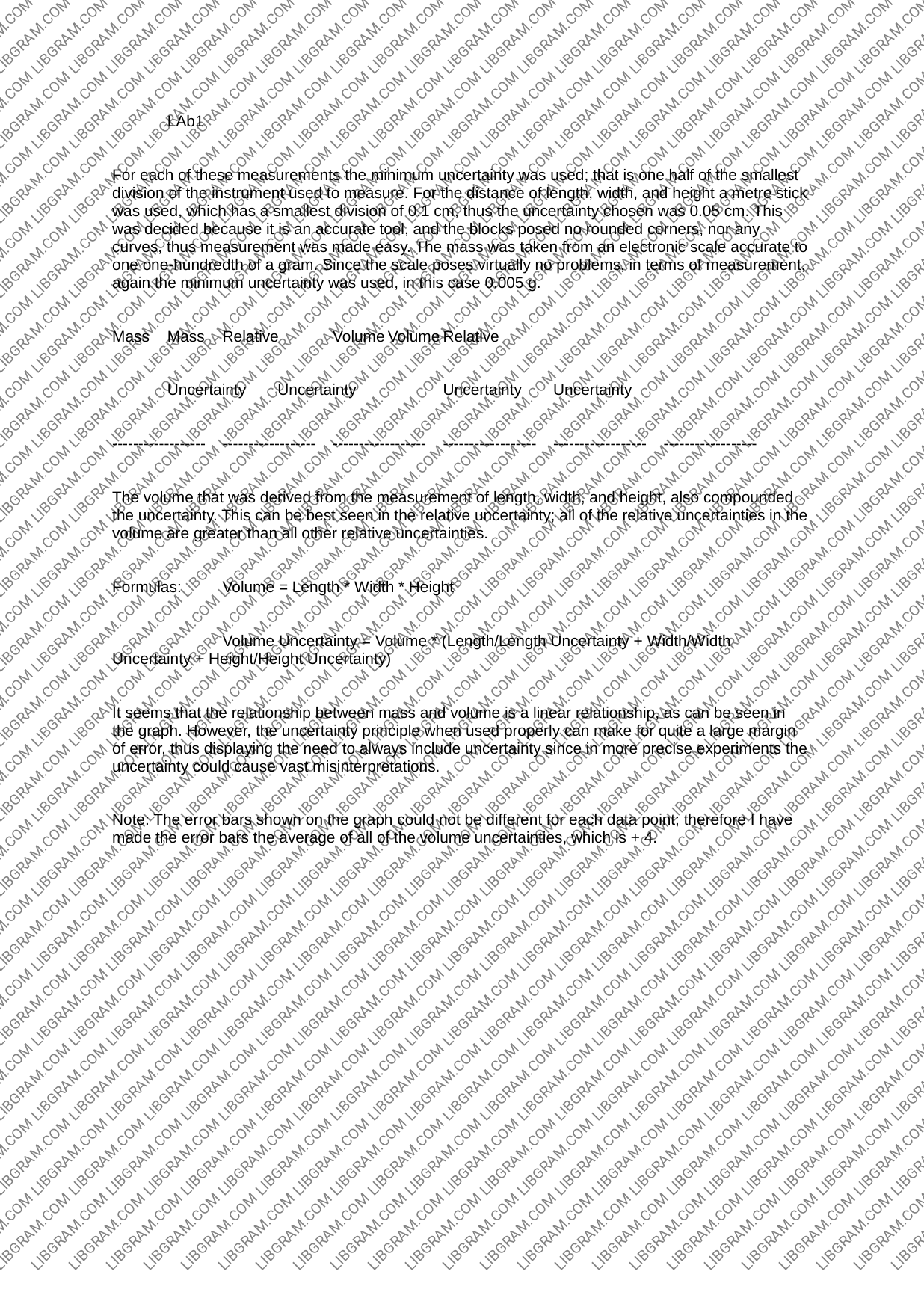# Paper: An Analysis of an Experiment on the Measurement of Length, Width and HeightLAb1 For each of these measurements the minimum uncertainty was used; that is one half of the smallest division of the instrument used to measure. For the distance of length, width, and height a metre stick was used, which has a smallest division of 0.1 cm, thus the uncertainty chosen was 0.05 cm. This was decided because it is an accurate tool, and the blocks posed no rounded corners, nor any curves, thus measurement was made easy. The mass was taken from an electronic scale accurate to one one-hundredth of a gram. Since the scale poses virtually no problems, in terms of measurement, again the minimum uncertainty was used, in this case 0.005 g. Mass Mass_ Relative Volume Volume Relative Uncertainty Uncertainty Uncertainty Uncertainty The volume that was derived from the measurement of length, width, and height, also compounded the uncertainty. This can be best seen in the relative uncertainty; all of the relative uncertainties in the volume are greater than all other relative uncertainties. Formulas: Volume = Length * Width * Height Volume Uncertainty = Volume * (Length/Length Uncertainty + Width/Width Uncertainty + Height/Height Uncertainty) It seems that the relationship between mass and volume is a linear relationship, as can be seen in the graph. However, the uncertainty principle when used properly can make for quite a large margin of error, thus displaying the need to always include uncertainty since in more precise experiments the uncertainty could cause vast misinterpretations. Note: The error bars shown on the graph could not be different for each data point; therefore | have made the error bars the average of all of the volume uncertainties, which is + 4. ...